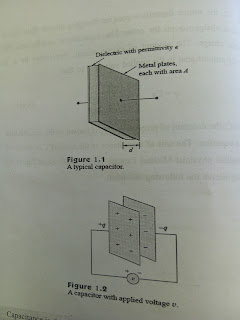# Introduction of energy Storage element (capacitor)

### INTRODUCTION of Energy Storage Element

So far we have limited our study to resistive circuits. we shall introduce two new and important passive linear circuit elements: the capacitor and the inductor. Unlike resistors, which dissipate energy
capacitors and inductors do not dissipate but store energy, which can be retrieved at a later time. For this reason, capacitors and inductors are
called storage elements.Typical capacitor

The application of resistive circuits is quite limited.With the introduction of capacitors and inductors in this chapter, we will be able to analyze
more important and practical circuits. Be assured that the circuit analysis techniques covered in Chapters 3 and 4 are equally appli cable to circuits with capacitors and inductors. We begin by introducing capacitors and describing how to com- bine them in series or in parallel. Later, we do the same for inductors. As typical applications, we explore how capacitors are combined with opampps to form integrators, differentiator  and analog computers.

#### Capacitors

A capacitor is a passive element designed to store energy in its clectric
field. Besides resistors, capacitors are the most common electrical
ponents. Capacitors are used extensively in electronics,
communications, computers, and power systems. For example, they are
used in the tuning circuits of radio receivers and as dynamic memory
elements in computer systems.
A capacitor is typically constructed as0depicted in Fig. 1.1com
A capacitor consists of two conducting plates separated by an(insulator (or dielectric)
In many practicalal applications, the plates may be aluminum foil while